# is Find an equation for, and identify, the surface that results when the surface x2 -...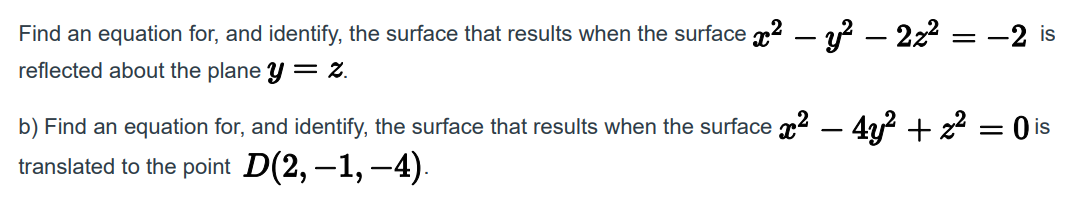is Find an equation for, and identify, the surface that results when the surface x2 - y2 – 2z2 reflected about the plane Y = 2. b) Find an equation for, and identify, the surface that results when the surface x2 – 4y2 + x2 = ( is translated to the point D(2,-1,-4).

The given equation can be rewritten as :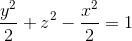which is the equation of a hyperboloid of one sheet along the x- axis, like one in the picture: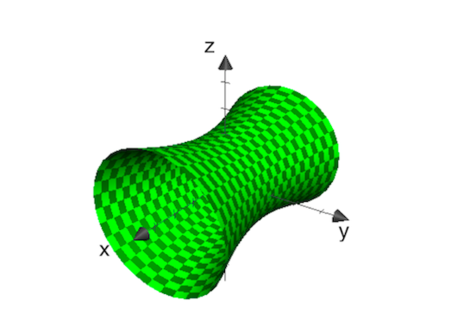Reflecting this surface about the plane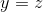will result in the same surface , since the surface is symmetric about the plane . Hence the resulting equation will be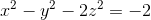or(b) The given equation can be rewritten as: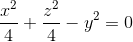which is the equation of an elliptic cone with axis y-axis. Translating the origin to D, we get the equation: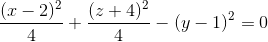which is the equation of an elliptic cone with centre D

##### Add Answer of: is Find an equation for, and identify, the surface that results when the surface x2 -...
Similar Homework Help Questions
• ### Exercise 3. Find and identify the trace of the given quadric surface in the specified plane...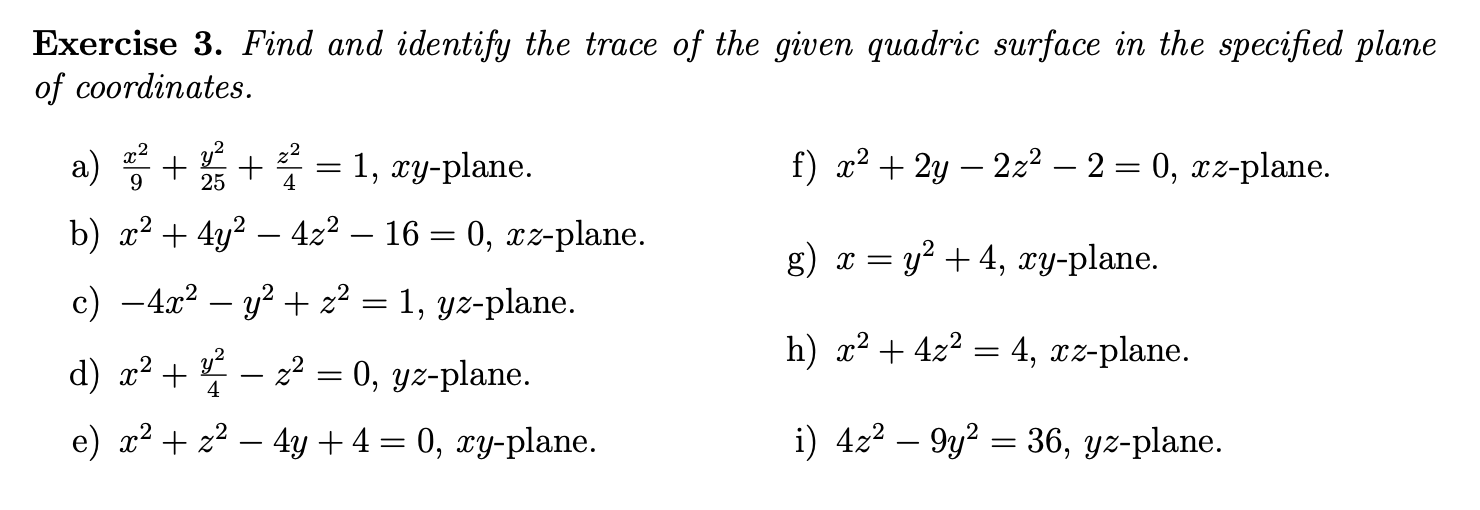Exercise 3. Find and identify the trace of the given quadric surface in the specified plane of coordinates. f) x2 + 2y – 2z2 – 2 = 0, xz-plane. g) x = y2 + 4, xy-plane. a) A + B + * = 1, xy-plane. b) x2 + 4y2 – 4z2 – 16 = 0, xz-plane. c) -4x2 - y2 + z2 = 1, yz-plane. d) x2 + – z2 = 0, yz-plane. e) x2 + x2 – 4y+4= 0,...

• ### (a) Find and identify the traces of the quadric surface x2 + y2 ? z2 =...

(a) Find and identify the traces of the quadric surface x2 + y2 ? z2 = 25 given the plane. x = k Find the trace. Identify the trace. y=k Find the trace. Identify the trace. z=k Find the trace Identify the trace. (b) If we change the equation in part (a) to x2 ? y2 + z2 = 25, how is the graph affected? (c) What if we change the equation in part (a) to x2 + y2 +...

• ### (a) Find and identify the traces of the quadric surface x2 + y2 − z2 =...

(a) Find and identify the traces of the quadric surface x2 + y2 − z2 = 4, given the plane. x = k y=k z=k (IDENTIFY TRACE AND SHAPE OF THE TRACE)

• ### (a) Find and identify the traces of the quadric surface x2 + y2 − z2 =...

(a) Find and identify the traces of the quadric surface x2 + y2 − z2 = 16 given the plane. x = k Find the trace. Identify the trace. circleellipse    hyperbolaparabola y = k Find the trace. Identify the trace. circleellipse    hyperbolaparabola z = k Find the trace. Identify the trace. circleellipse    hyperbolaparabola Describe the surface from one of the graphs in the table. ellipsoidelliptic paraboloid    hyperbolic paraboloidconehyperboloid of one sheethyperboloid of two sheets (b) If we change the equation in part (a) to...

• ### 1) Consider the surface x2 + 3y2-2z2-1 (a) What are the cross sections(traces) in x k,y k, z k Sketch for (b) Sketch th...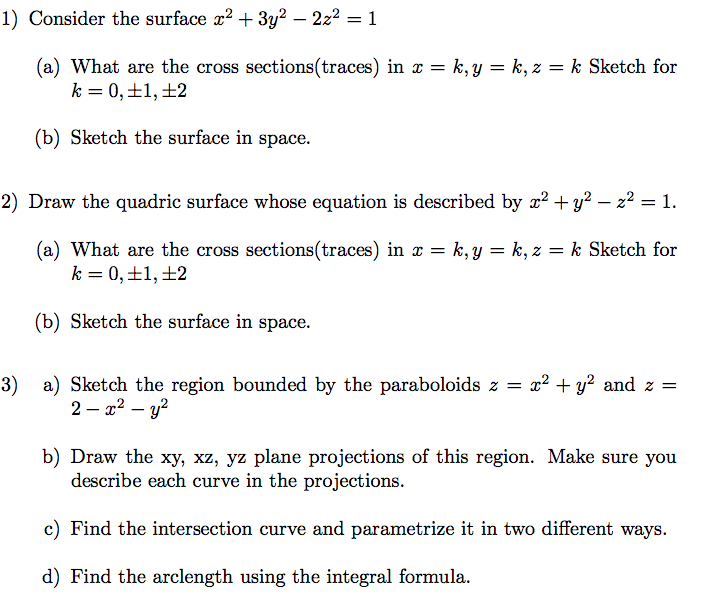1) Consider the surface x2 + 3y2-2z2-1 (a) What are the cross sections(traces) in x k,y k, z k Sketch for (b) Sketch the surface in space. 2) Draw the quadric surface whose equation is described by z2 +y2 - 221 (a) What are the cross sections(traces) inx-k,y k,z k Sketch for (b) Sketch the surface in space. a) Sketch the region bounded by the paraboloids z-22 + y2 and z - 3) 2 b) Draw the xy, xz, yz...

• ### find an equation of the tangent plane to surface

Find an equation of the tangent plane to the surface at the givenpoint.z = x2 - 2xy +y2, (4, 5, 1)x + y + z + = 0

• ### Find the equation of the plane tangent to the following surface at the given points. x2...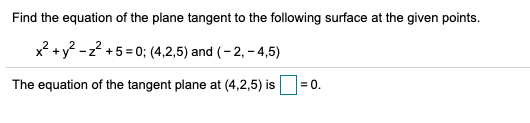Find the equation of the plane tangent to the following surface at the given points. x2 + y2 - 2? + 5 = 0; (4,2,5) and (-2,-4,5) The equation of the tangent plane at (4,2,5) is = 0. the equation of the tangent plane to the surface

• ### Identify the surface 4.x2 - y2 + 2z2 +4 = 0. Hyperboloid of one sheets Elliptic...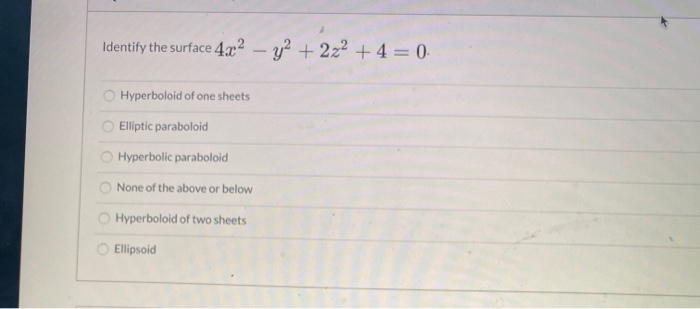Identify the surface 4.x2 - y2 + 2z2 +4 = 0. Hyperboloid of one sheets Elliptic paraboloid Hyperbolic paraboloid None of the above or below Hyperboloid of two sheets Ellipsoid

• ### (a) Find and identify the traces of the quadric surface x2 + y2 ? z2 =...

(a) Find and identify the traces of the quadric surface x2 + y2 ? z2 = 16 given the plane. x = k Find the trace. y = k Find the trace. z = k Find the trace. Describe the surface from one of the graphs in the table. ellipsoid elliptic paraboloid hyperbolic paraboloid cone hyperboloid of one sheet hyperboloid of two sheets

• ### 1. Consider the surface of revolution that is given by the equation Z-R= -(x2 + y2)/R...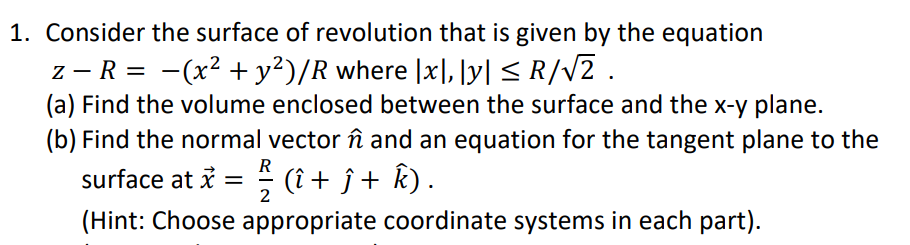1. Consider the surface of revolution that is given by the equation Z-R= -(x2 + y2)/R where [x],[y] < R/V2 . (a) Find the volume enclosed between the surface and the x-y plane. (b) Find the normal vector în and an equation for the tangent plane to the surface at i = ? (î+ ſ + Â). (Hint: Choose appropriate coordinate systems in each part).

Free Homework App